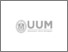UUM Electronic Theses and Dissertation
UUM ETD | Universiti Utara Malaysian Electronic Theses and Dissertation
FAQs | Feedback | Search Tips | Sitemap

# New spline methods for solving first and second order ordinary differential equations

Ala'yed, Osama Hasan Salman (2016) New spline methods for solving first and second order ordinary differential equations. PhD. thesis, Universiti Utara Malaysia.Preview
Text
s95069_01.pdfPreview
Text
s95069_02.pdf

## Abstract

Many problems arise from various real life applications may lead to mathematical models which can be expressed as initial value problems (IVPs) and boundary value problems (BVPs) of first and second ordinary differential equations (ODEs).These
problems might not have analytical solutions, thus numerical methods are needed inapproximating the solutions. When a differential equation is solved numerically, the interval of integration is divided into subintervals.Consequently, numerical solutions at the grid pointscan be determined through numerical computations, whereas the solutions between the grid points still remain unknown. In order to find the approximate solutions between any two grid points, spline methods are introduced. However, most of the existing spline methods are used to approximate the solutions of specific cases of IVPs and BVPs. Therefore, this study develops new spline methods based on polynomial and non-polynomial spline functions for solving general cases of first and second order IVPs and BVPs. The convergence analysis for each new spline method is also discussed. In terms of implementation, the
4-stage fourth order explicit Runge-Kutta method is employed to obtain the solutions at the grid points, while the new spline methods are used to obtain the solutions between the grid points. The performance of the new spline methods are then compared with the existing spline methods in solving12 test problems. Generally, the numerical results indicate that the new spline methods provide better accuracy than their counterparts. Hence, the new spline methods are viable
alternatives for solving first and second order IVPs and BVPs.

Item Type: Thesis (PhD.) Teh, Yuan Ying and Saaban, Azizan 6037 Interpolation, Spline method, Initial value problem, Boundary value problem, Ordinary differential equation. Q Science > QA Mathematics Awang Had Salleh Graduate School of Arts & Sciences 07 Feb 2017 10:34 05 Apr 2021 01:51 Awang Had Salleh Graduate School of Arts and Sciences Teh, Yuan Ying and Saaban, Azizan https://etd.uum.edu.my/id/eprint/6037